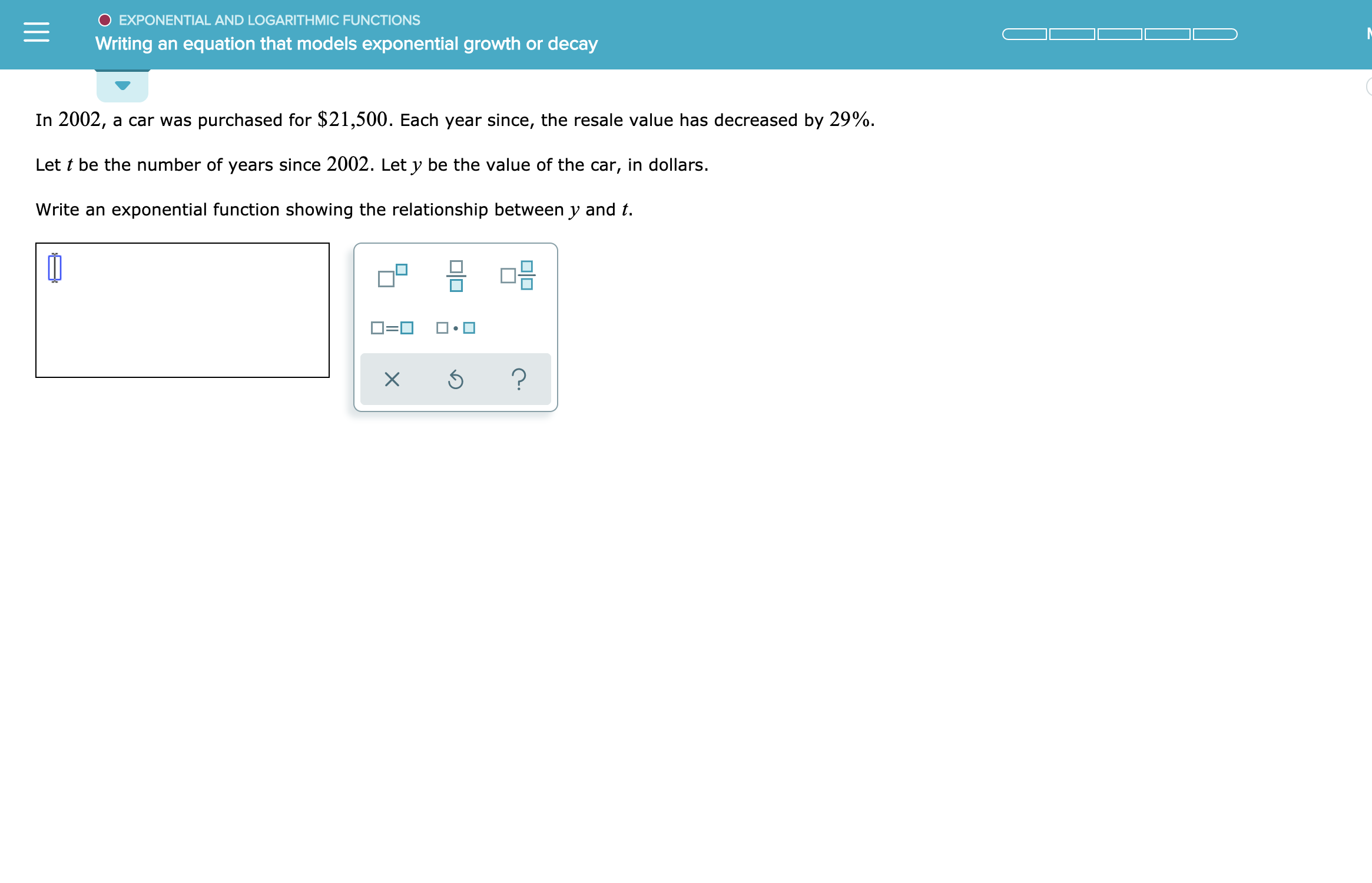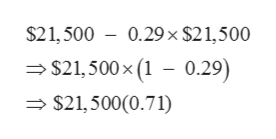EXPONENTIAL AND LOGARITHMIC FUNCTIONSWriting an equation that models exponential growth or decayIn 2002a car was purchased for \$21,500. Each year since, the resale value has decreased by 29%.Let t be the number of years since 2002. Let y be the value of the car, in dollars.Write an exponential function showing the relationship between y and tO=O?

Question

See attachmenthelp_outlineImage TranscriptioncloseEXPONENTIAL AND LOGARITHMIC FUNCTIONS Writing an equation that models exponential growth or decay In 2002 a car was purchased for \$21,500. Each year since, the resale value has decreased by 29%. Let t be the number of years since 2002. Let y be the value of the car, in dollars. Write an exponential function showing the relationship between y and t O=O ? fullscreen
Step 1

given that cost price of car is \$21500

and rate of depreciation is 29% per year

number of years = t

Value of car is y

Step 2

To write an exponential function showing relationship between y and t

Step 3

Original Price = \$21,500

if reduced by 29% each year,

af...help_outlineImage Transcriptionclose\$21,500 0.29x \$21,500 \$21,500x (1 - 0.29) \$21,500(0.71) fullscreen

Want to see the full answer?

See Solution

Want to see this answer and more?

Our solutions are written by experts, many with advanced degrees, and available 24/7

See Solution
Tagged in

Other